# The SSM Procedure

#### Multivariate Season

The STATE statement option TYPE=SEASON(LENGTH=s) specifies a ((s–1)*dim)-dimensional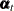, needed for defining a dim-dimensional trigonometric season component with season length s. A (multivariate) trigonometric season component,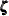, is a sum of (multivariate) cycles of different frequencies,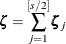where the constituent cycles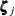, called harmonics, have frequencies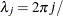s. All the harmonics are assumed to be statistically independent, have the same damping factor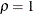, and are governed by the disturbances with the same covariance matrix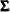. The number of harmonics,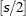, equals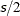if s is even and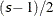if it is odd. This means that specifying TYPE=SEASON(LENGTH=s) is equivalent to specifyingcycle specifications with correct frequencies, damping factor, and the COV option restricted to the same covariance. The resultingis necessarily ((s–1)*dim)-dimensional. When the season lengthis even, the last harmonic cycle,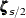, has frequency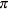and requires special attention. It is of dimension dim rather than 2*dim because its underlying state equation simplifies to a dim-variate autoregression with autoregression coefficient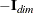. As a result of this discussion, it is clear that the system matrices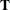and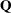associated with the ((s–1)*dim)-dimensionalare block-diagonal with the blocks corresponding to the harmonics. The initial condition is fully diffuse.

For all the models discussed so far, the first dim elements ofprovided the needed (multivariate) component. This is not the case for the (multivariate) season component. Extracting the ith seasonal component fromrequires accumulating the contributions from theharmonics that are associated with this ith seasonal, which are not organized contiguously in. For example, suppose that dim is 2 and the season length s is 4. In this caseis 2, and the bivariate seasonal component is a sum of two independent bivariate cycles,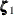and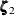. The cyclehas frequency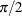and its underlying state, say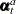, has dimension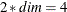. The last harmonic,, has frequency, and therefore its underlying state, say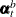, has dimension 2. The combined state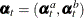has dimension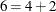. In order to extract the first bivariate seasonal component, you must extract the first components of bivariate cyclesand, which in turn implies the first elements ofand, respectively. Thus, obtaining the first bivariate seasonal component requires extracting the first and the fifth elements of the combined state. Similarly, obtaining the second bivariate seasonal component requires extracting the second and the sixth elements of the combined state. All this can be summarized by the dot product expressions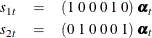where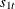and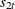denote the first and second components, respectively, of the bivariate seasonal component. Note thatandare univariate seasonal components, each of season length 4, in their own right. They are correlated components; their correlation structure depends on.

Obtaining the desired components of the multivariate seasonal component is made easy by a special syntax convention of the COMPONENT statement. Continuing with the previous example, the following examples illustrate two equivalent ways of obtainingand. The first set of statements explicitly specify the linear combinations needed for definingand:

     state seasonState(2) type=season(length=4)  ...;
component s_1 =( 1  0  0  0  1  0 ) * seasonState;
component s_2 =( 0  1  0  0  0  1 ) * seasonState;


The following simpler specification achieves the same result:

     state seasonState(2) type=season(length=4)  ...;
component s_1 = seasonState;
component s_2 = seasonState;


In the latter specification, the meaning of the element operator [] changes if the state in question is defined by using the TYPE= option.